R packages:

# Install necessary packages
list_packages <- c("forecast", "readxl", "stargazer", "fpp",
"fpp2", "scales", "quantmod", "urca",
"vars", "tseries", "ggplot2", "dplyr")
new_packages <- list_packages[!(list_packages %in% installed.packages()[,"Package"])]
if(length(new_packages)) install.packages(new_packages)

lapply(list_packages, require, character.only = TRUE)
Model ACF, $$\rho_k$$ PACF, $$\phi_{kk}$$
AR(p) exponentially decay cut off at lag $$p$$
MA(q) cut off at lag $$q$$ exponentially decay
ARMA(p,q) exponentially decay after lag $$q$$ exponentially decay
ARIMA(p,d,q) slowly decrease exponentially decay

# 1 Identification for Nonstationary Models

To identify the nonstationarity, you can

• plot the time series, look for changes in mean or variance.
• look at the ACF to see if the ACF decreases slowly.
• look at the parameter estimate, see if the estimated parameters are outside of stationarity region, e.g., $$|\phi_1|<1$$ for AR(1).
• The last two points are a little arbitrary, so we use augmented Dickey-Fuller test (ADF).

Null hypothesis, $$H_0$$: There is a unit root, i.e., homogeneous nonstationarity.

Alternative hypothesis, $$H_1$$: There is no unit root, i.e., stationarity.

Therefore, small p-value indicates stationarity and large p-value indicates homogeneous nonstationarity, i.e., there is a unit root.

The test statistic has a special distribution that’s not commonly seen, so it make more sense to just look at the p-value.

Case 1:

case1=as.ts(scan("case1.txt"))
par(mfrow=c(1,3))
plot(case1)
acf(case1)
pacf(case1)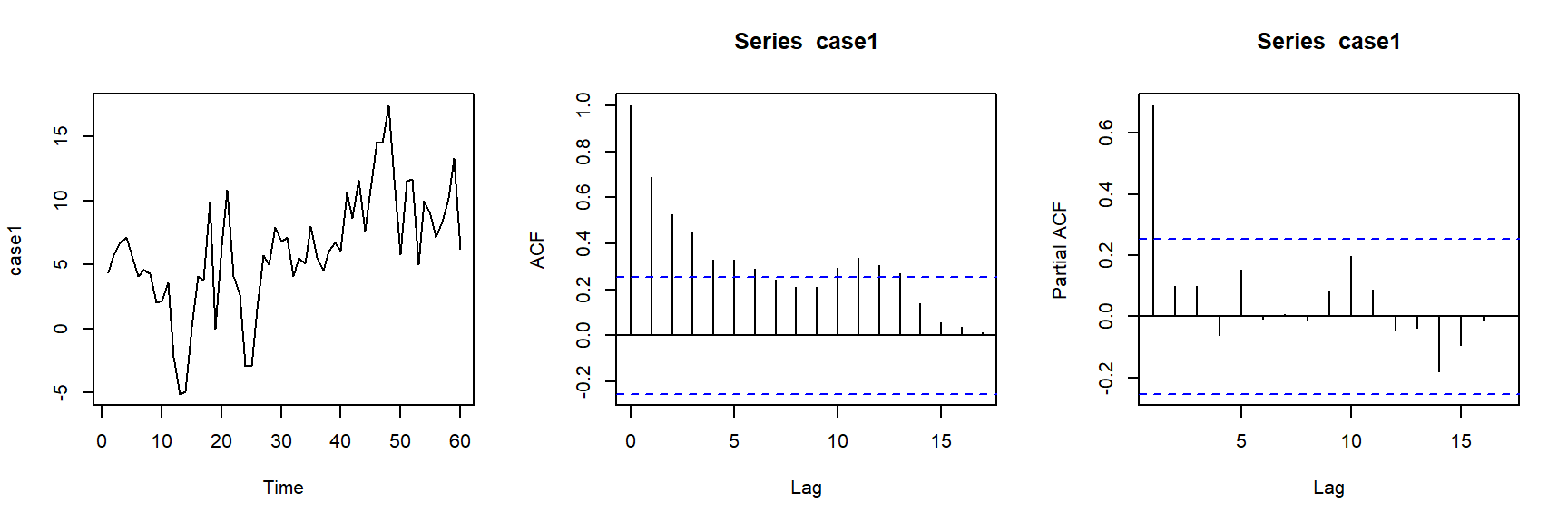adf.test(case1)
##
##  Augmented Dickey-Fuller Test
##
## data:  case1
## Dickey-Fuller = -3.6119, Lag order = 3, p-value = 0.0398
## alternative hypothesis: stationary

# 2 Nonstationarity Tests and Their Basic Ideas

## 2.1 Dickey-Fuller Test

To illustrate Dickey-Fuller test, let’s take AR(1) as an example, we have

$Z_t - \mu = \phi_1 (Z_{t-1} - \mu) + a_t\\ Z_t=C + \phi_1 Z_{t-1} + a_t$ where $$C=(1-\phi_1)\mu$$. Sometimes, we allow the mean of the time series to be a linear function in time, that is,

$Z_t=C + \beta t + \phi_1 Z_{t-1} + a_t \\ Z_t - \frac{C + \beta t}{1-\phi_1} = \phi_1 \left(Z_{t-1} - \frac{C + \beta t}{1-\phi_1} \right) + a_t$

Below is an example of the AR(1) for $$C=0$$ vs $$C \neq 0$$, $$\beta=0$$ vs $$\beta \neq 0$$, and $$\phi_1=1$$ vs $$\phi_1 < 1$$.

par(mfrow=c(3,2))
N <- 500
a <- 1
l <- 0.01
rho <- 0.7

set.seed(123)
v <- ts(rnorm(N,0,1))

y <- ts(rep(0,N))
for (t in 2:N){
y[t]<- rho*y[t-1]+v[t]
}
plot(y,type='l', ylab="phi*Z[t-1] + a[t]")
abline(h=0)

y <- ts(rep(0,N))
for (t in 2:N){
y[t]<- y[t-1]+v[t]
}
plot(y,type='l', ylab="Z[t-1] + a[t]")
abline(h=0)

y <- ts(rep(0,N))
for (t in 2:N){
y[t]<- a+rho*y[t-1]+v[t]
}
plot(y,type='l', ylab="C + phi*Z[t-1]+a[t]")
abline(h=0)

a <- 0.1
y <- ts(rep(0,N))
for (t in 2:N){
y[t]<- a+y[t-1]+v[t]
}
plot(y,type='l', ylab="C + Z[t-1] + a[t]")
abline(h=0)

y <- ts(rep(0,N))
for (t in 2:N){
y[t]<- a+l*time(y)[t]+rho*y[t-1]+v[t]
}
plot(y,type='l', ylab="C + beta*t + phi*Z[t-1] + a[t]")
abline(h=0)

y <- ts(rep(0,N))
for (t in 2:N){
y[t]<- a+l*time(y)[t]+y[t-1]+v[t]
}
plot(y,type='l', ylab="C + beta*t + Z[t-1] + a[t]")
abline(h=0)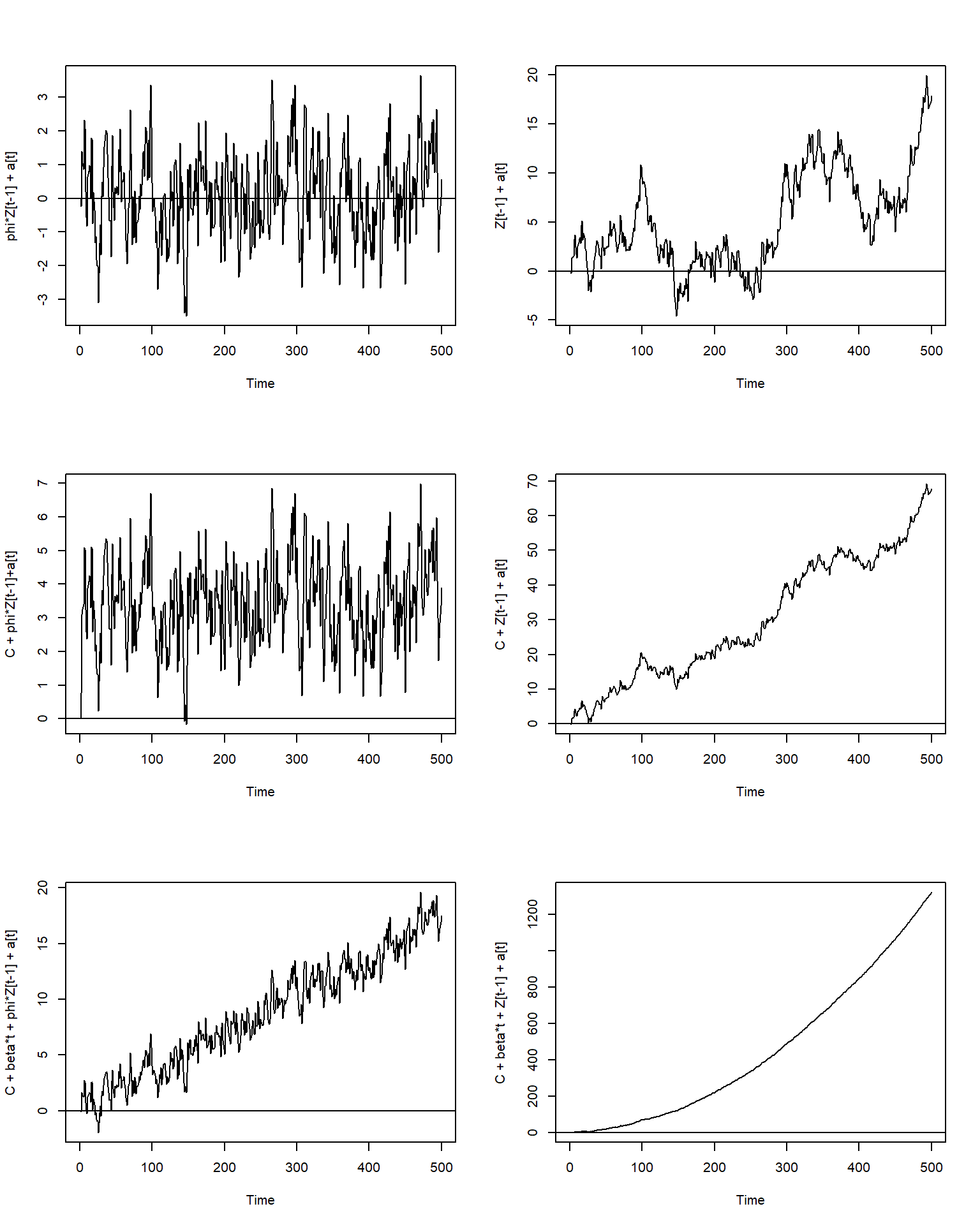With a little algebra, it becomes

$Z_t = C + \beta t + \phi_1 Z_{t-1} + a_t \\ Z_t - Z_{t-1} = C + \beta t + (\phi_1 - 1) Z_{t-1} + a_t\\ \nabla Z_t = C + \beta t + \gamma Z_{t-1} + a_t$

If $$\gamma=0$$, then we have a random walk process. If not and $$-1<\gamma+1<1$$, then we have a stationary process.

## 2.2 Augmented Dickey-Fuller Test

The Augmented Dickey-Fuller test allows for higher-order autoregressive processes. Let’s take AR(2) as an example.

$Z_t = C + \beta t + \phi_1 Z_{t-1} + \phi_2 Z_{t-2} + a_t \\ Z_t - Z_{t-1} = C + \beta t + (\phi_1 + \phi_2 - 1) Z_{t-1} - \phi_2 (Z_{t-1} - Z_{t-2}) + a_t\\ \nabla Z_t = C + \beta t + \gamma Z_{t-1} - \phi_2 \nabla Z_{t-1} + a_t$ where $$\gamma=(\phi_1 + ... + \phi_p - 1)$$.

If this AR(2) is homogeneous nonstationary (i.e., has a unit root), then root of the AR polynomial $$1-\phi_1 B - \phi_2 B^2=0$$ has a unit root $$B=1$$. If we plug in $$B=1$$ in $$1-\phi_1 B - \phi_2 B^2=0$$, we have $$1-\phi_1 1 - \phi_2 1^2=1-\phi_1 - \phi_2 =0$$. So testing the unit root is equivalent to test the regression coefficient $$\gamma=0$$ or not. Therefore, we can simply run regress $$\nabla Z_t$$ on $$Z_{t-1}$$, $$\nabla Z_{t-1}$$, $$t$$ and intercept. Then test $$\gamma=0$$ using $$t=\hat{\gamma}/SE(\hat{\gamma})$$.

However, $$t=\hat{\gamma}/SE(\hat{\gamma})$$ from the regression does not follow a t distribution. Dickey an Fuller develop the distribution of $$t=\hat{\gamma}/SE(\hat{\gamma})$$ in their 1979 and 1981 paper.

However, note that this is a one tail test. We reject the null hypothesis when $$\hat{\gamma}$$ is small or negative, because $$\gamma > 0$$ implies $$1-\phi_1 - \phi_2 < 0$$ which is stationarity.

If $$t=\hat{\gamma}/SE(\hat{\gamma}) < -2.86$$, then reject $$H_0$$, which implies stationarity. Or we can look at the p-value from the output.

To be effective in ADF test, the test requires that the AR part of the model be correctly specified.

It can be proved that if all roots of $$1-\phi_1 B - \phi_2 B^2=0$$ are beyond 1 in absolute value, then

$\phi_1+\phi_2<1\\ \phi_2-\phi_1<1\\ -1<\phi_2<1$

which is equivalent to

$1 - \phi_1 - \phi_2 >0\\ 1 + \phi_1 - \phi_2 >0\\ -1<\phi_2<1$

In other words, the pair $$(\phi_1, \phi_2)$$ has to be inside of the triangle below in order to have stationary AR(2).

plot(0,0,type="n",xlab="phi_1",ylab="phi_2",xlim=c(-3,3),ylim=c(-2,2))
abline(h=0,col="grey")
abline(v=0,col="grey")
lines(c(0,2),c(1,-1))
lines(c(0,-2),c(1,-1))
lines(c(-2,2),c(-1,-1))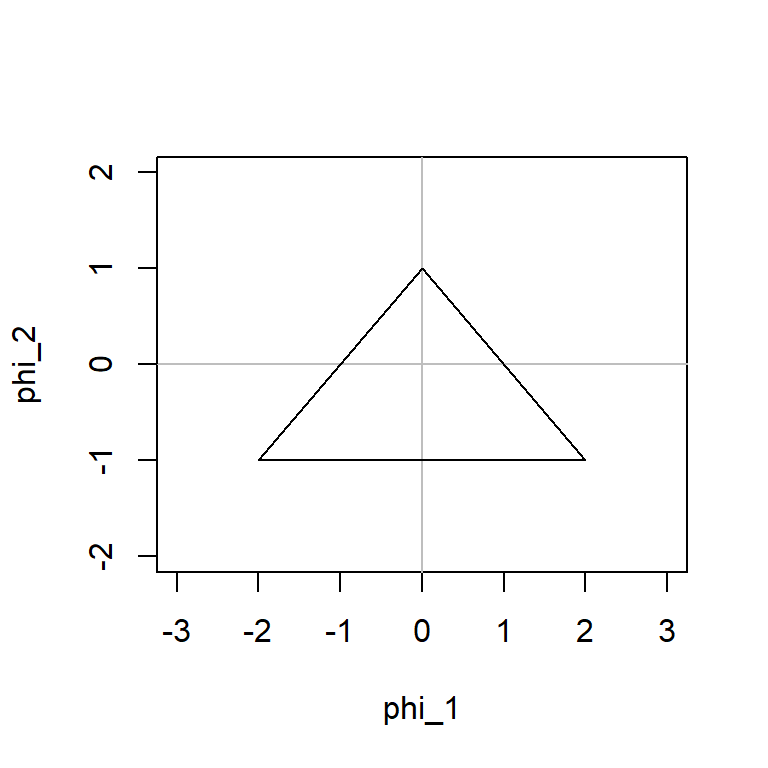The approximate 5% left tail critical value is -2.86.

Three cases are considered

• No mean in the model, i.e., $$\mu=0$$.

• A constant mean in the model, i.e., $$\mu \neq 0$$.

• A linear trend in the model, i.e., $$\mu = a + b t$$.

case 1 above may be reasonable if you have already differenced your original time series data. i.e., $$Z_t - Z_{t-1}$$ usually has a zero mean if $$Z_t$$, $$Z_{t-1}$$ have the same constant mean.

But if the original time series data had a deterministic trend $$\alpha + \beta t$$, then differencing induces a constant mean.

# 3 Examples of Nonstationarity Tests

The adf.test() from the tseries package will do an Augmented Dickey-Fuller test (or Dickey-Fuller if we set lags equal to 0) with a trend $$\beta t$$ and an intercept $$C$$.

The ur.df() in the urca package also conducts an Augmented Dickey-Fuller test and gives us a bit more information and control over the test. The ur.df() function allows us to specify whether to test stationarity around a zero-mean with no trend ($$C=0$$ and $$\beta=0$$), around a non-zero mean with no trend ($$C \neq 0$$ and $$\beta=0$$), or around a trend with an intercept ($$C \neq 0$$ and $$\beta \neq 0$$). This can be useful when we know that our data have no trend, for example if you have removed the trend already. ur.df() allows us to specify the lags or select them using model selection. If type is set to "none" neither an intercept nor a trend is included in the test regression. If it is set to "drift" an intercept is added and if it is set to "trend" both an intercept and a trend is added. For this test, the more negative the test statistic is, the stronger the rejection of the hypothesis that there is a unit root at some level of confidence.

The ur.kpss() function from the urca package performs the Kwiatkowski-Phillips-Schmidt-Shin (KPSS) test, which is similar to ADF test. In the KPSS test, the null hypothesis is that the data are stationary, and we look for evidence that the null hypothesis is false. Small p-values (e.g., less than 0.05) suggest that differencing is required.

Case 5: rail freight

case5=as.ts(scan("case5.txt"))
par(mfrow=c(1,3))
plot(case5)
acf(case5)
pacf(case5)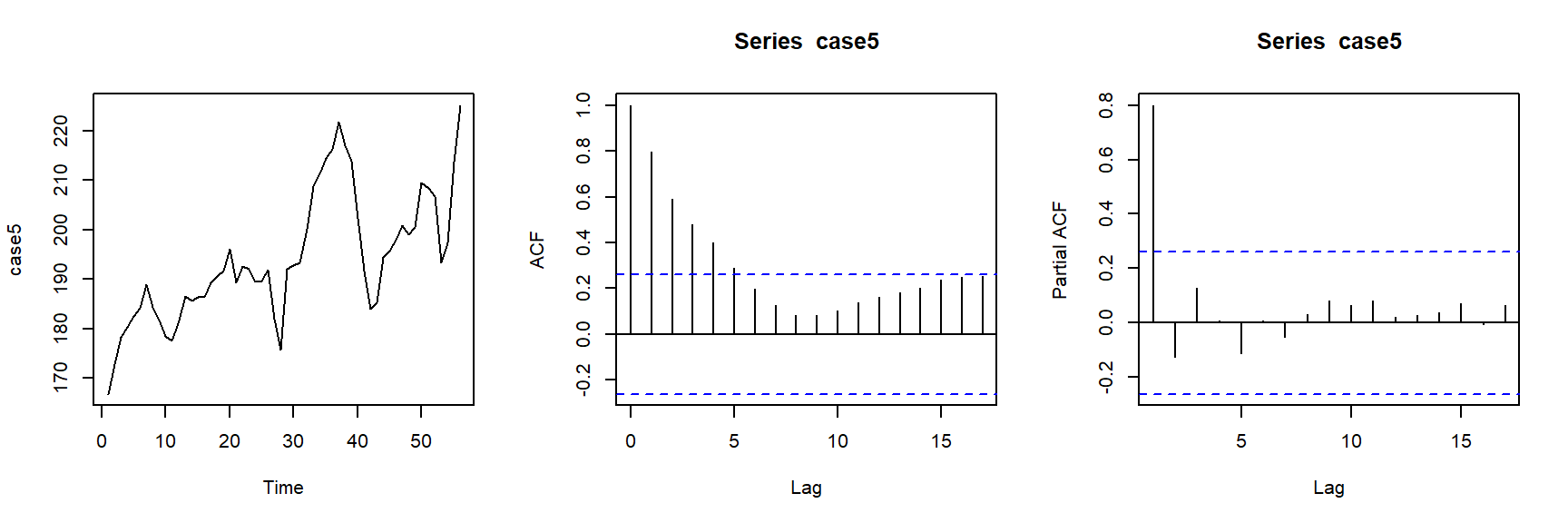adf.test(case5,k=1)
##
##  Augmented Dickey-Fuller Test
##
## data:  case5
## Dickey-Fuller = -3.368, Lag order = 1, p-value = 0.06997
## alternative hypothesis: stationary
adf.test(case5,k=2)
##
##  Augmented Dickey-Fuller Test
##
## data:  case5
## Dickey-Fuller = -2.9204, Lag order = 2, p-value = 0.2035
## alternative hypothesis: stationary
adf.test(case5,k=3)
##
##  Augmented Dickey-Fuller Test
##
## data:  case5
## Dickey-Fuller = -3.051, Lag order = 3, p-value = 0.1508
## alternative hypothesis: stationary

The ADF test suggest not reject the null hypothesis, that is, the time series is nonstationary.

test=ur.df(case5, type = "trend", lags = 3)
summary(test)
##
## ###############################################
## # Augmented Dickey-Fuller Test Unit Root Test #
## ###############################################
##
## Test regression trend
##
##
## Call:
## lm(formula = z.diff ~ z.lag.1 + 1 + tt + z.diff.lag)
##
## Residuals:
##      Min       1Q   Median       3Q      Max
## -14.9846  -3.0991   0.0496   3.9368  12.2649
##
## Coefficients:
##             Estimate Std. Error t value Pr(>|t|)
## (Intercept) 59.69577   19.61985   3.043  0.00387 **
## z.lag.1     -0.33522    0.10987  -3.051  0.00378 **
## tt           0.20327    0.07839   2.593  0.01271 *
## z.diff.lag1  0.47177    0.14597   3.232  0.00228 **
## z.diff.lag2 -0.01762    0.15835  -0.111  0.91189
## z.diff.lag3  0.15639    0.15690   0.997  0.32411
## ---
## Signif. codes:  0 '***' 0.001 '**' 0.01 '*' 0.05 '.' 0.1 ' ' 1
##
## Residual standard error: 5.497 on 46 degrees of freedom
## Multiple R-squared:  0.2598, Adjusted R-squared:  0.1794
## F-statistic: 3.229 on 5 and 46 DF,  p-value: 0.01396
##
##
## Value of test-statistic is: -3.051 3.491 4.7557
##
## Critical values for test statistics:
##       1pct  5pct 10pct
## tau3 -4.04 -3.45 -3.15
## phi2  6.50  4.88  4.16
## phi3  8.73  6.49  5.47

We only look at the first of the three statistics. The other two are dealing with uncertainty about including the intercept and deterministic time trend terms in the test equation.

The ADF test above suggests that we cannot reject the null hypothesis and conclude the time series data is nonstationary.

test=ur.df(case5, type = "drift", lags = 3)
summary(test)
##
## ###############################################
## # Augmented Dickey-Fuller Test Unit Root Test #
## ###############################################
##
## Test regression drift
##
##
## Call:
## lm(formula = z.diff ~ z.lag.1 + 1 + z.diff.lag)
##
## Residuals:
##      Min       1Q   Median       3Q      Max
## -12.2334  -3.1932   0.2355   2.6387  14.1785
##
## Coefficients:
##             Estimate Std. Error t value Pr(>|t|)
## (Intercept) 24.17319   14.87568   1.625   0.1108
## z.lag.1     -0.12078    0.07661  -1.576   0.1216
## z.diff.lag1  0.37208    0.14914   2.495   0.0162 *
## z.diff.lag2 -0.15053    0.15868  -0.949   0.3477
## z.diff.lag3  0.01032    0.15510   0.067   0.9472
## ---
## Signif. codes:  0 '***' 0.001 '**' 0.01 '*' 0.05 '.' 0.1 ' ' 1
##
## Residual standard error: 5.822 on 47 degrees of freedom
## Multiple R-squared:  0.1516, Adjusted R-squared:  0.07942
## F-statistic:   2.1 on 4 and 47 DF,  p-value: 0.09574
##
##
## Value of test-statistic is: -1.5765 1.6712
##
## Critical values for test statistics:
##       1pct  5pct 10pct
## tau2 -3.51 -2.89 -2.58
## phi1  6.70  4.71  3.86

We only look at the first of the two statistics. The other is dealing with uncertainty about including the intercept term in the test equation.

test=ur.df(case5, type = "none", lags = 3)
summary(test)
##
## ###############################################
## # Augmented Dickey-Fuller Test Unit Root Test #
## ###############################################
##
## Test regression none
##
##
## Call:
## lm(formula = z.diff ~ z.lag.1 - 1 + z.diff.lag)
##
## Residuals:
##      Min       1Q   Median       3Q      Max
## -13.5791  -3.1291   0.6174   3.4032  15.7122
##
## Coefficients:
##              Estimate Std. Error t value Pr(>|t|)
## z.lag.1      0.003531   0.004287   0.824   0.4142
## z.diff.lag1  0.311087   0.146789   2.119   0.0393 *
## z.diff.lag2 -0.200733   0.158285  -1.268   0.2109
## z.diff.lag3 -0.027277   0.155963  -0.175   0.8619
## ---
## Signif. codes:  0 '***' 0.001 '**' 0.01 '*' 0.05 '.' 0.1 ' ' 1
##
## Residual standard error: 5.921 on 48 degrees of freedom
## Multiple R-squared:  0.122,  Adjusted R-squared:  0.04884
## F-statistic: 1.668 on 4 and 48 DF,  p-value: 0.173
##
##
## Value of test-statistic is: 0.8237
##
## Critical values for test statistics:
##      1pct  5pct 10pct
## tau1 -2.6 -1.95 -1.61

There is only one test statistic.

test=ur.kpss(case5)
summary(test)
##
## #######################
## # KPSS Unit Root Test #
## #######################
##
## Test is of type: mu with 3 lags.
##
## Value of test-statistic is: 0.9756
##
## Critical value for a significance level of:
##                 10pct  5pct 2.5pct  1pct
## critical values 0.347 0.463  0.574 0.739

The KPSS test above suggests that we can reject the null hypothesis ($$H_0$$: stationary) and conclude the time series data is nonstationary.

Case IMA: refreigerator

d=scan("case_refrigerator.txt")
case_ref=as.ts(d[seq(2,length(d),2)])
par(mfrow=c(1,3))
plot(case_ref)
acf(case_ref)
pacf(case_ref)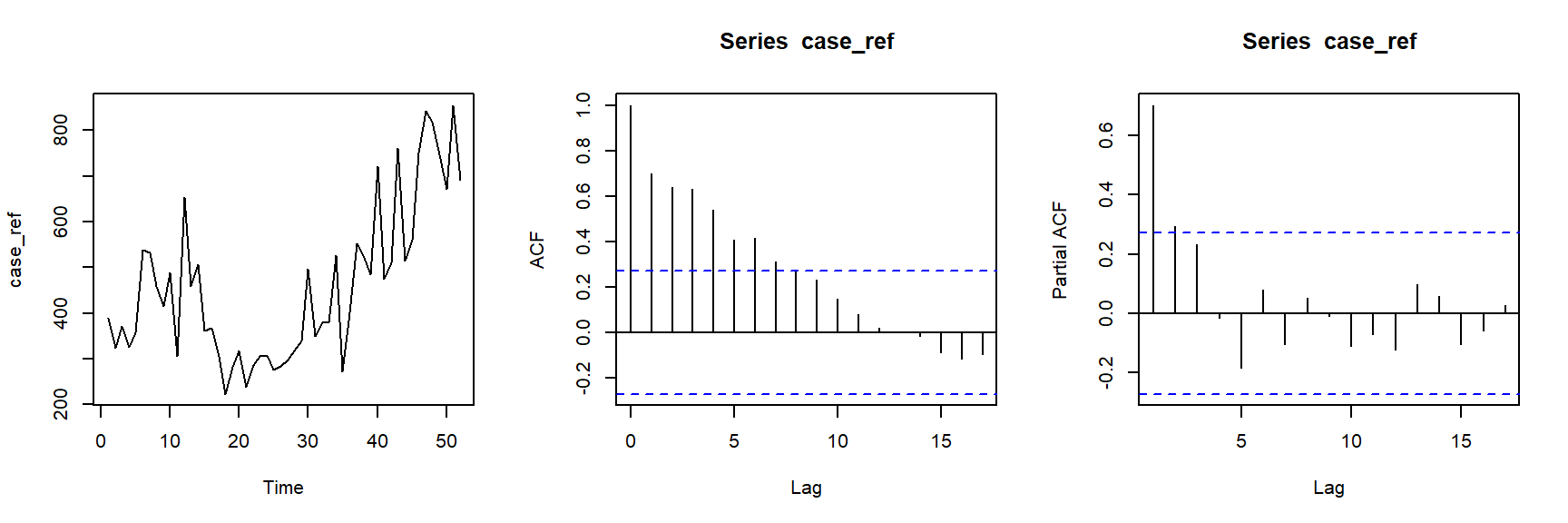adf.test(case_ref)
##
##  Augmented Dickey-Fuller Test
##
## data:  case_ref
## Dickey-Fuller = -1.1373, Lag order = 3, p-value = 0.9083
## alternative hypothesis: stationary
test=ur.df(case_ref, type = "trend", lags = 3)
summary(test)
##
## ###############################################
## # Augmented Dickey-Fuller Test Unit Root Test #
## ###############################################
##
## Test regression trend
##
##
## Call:
## lm(formula = z.diff ~ z.lag.1 + 1 + tt + z.diff.lag)
##
## Residuals:
##     Min      1Q  Median      3Q     Max
## -182.38  -64.51  -17.86   71.50  252.94
##
## Coefficients:
##             Estimate Std. Error t value Pr(>|t|)
## (Intercept) 35.74762   53.10076   0.673  0.50451
## z.lag.1     -0.16163    0.14212  -1.137  0.26186
## tt           2.03732    1.45829   1.397  0.16973
## z.diff.lag1 -0.60557    0.19037  -3.181  0.00276 **
## z.diff.lag2 -0.38260    0.19095  -2.004  0.05159 .
## z.diff.lag3 -0.09621    0.15945  -0.603  0.54950
## ---
## Signif. codes:  0 '***' 0.001 '**' 0.01 '*' 0.05 '.' 0.1 ' ' 1
##
## Residual standard error: 110.1 on 42 degrees of freedom
## Multiple R-squared:  0.3803, Adjusted R-squared:  0.3066
## F-statistic: 5.156 on 5 and 42 DF,  p-value: 0.0008912
##
##
## Value of test-statistic is: -1.1373 1.1539 1.0465
##
## Critical values for test statistics:
##       1pct  5pct 10pct
## tau3 -4.04 -3.45 -3.15
## phi2  6.50  4.88  4.16
## phi3  8.73  6.49  5.47
test=ur.kpss(case_ref)
summary(test)
##
## #######################
## # KPSS Unit Root Test #
## #######################
##
## Test is of type: mu with 3 lags.
##
## Value of test-statistic is: 0.7586
##
## Critical value for a significance level of:
##                 10pct  5pct 2.5pct  1pct
## critical values 0.347 0.463  0.574 0.739

# 4 ARIMA Models Equivalence

An ARIMA model can be written as

$(1-\phi_1 B - ... - \phi_p B^p) (1-B)^d Z_t = C + (1-\theta_1 B - ... - \theta_q B^q) a_t$

which is equivalent to

$(1-\phi_1 B - ... - \phi_p B^p) (1-B)^d \left(Z_t - \frac{\mu t^d}{d!}\right) = (1-\theta_1 B - ... - \theta_q B^q) a_t$

where $$C= \mu (1-\phi_1...-\phi_p)$$ and $$\mu$$ is the mean of $$(1-B)^d Z_t$$. R uses the second notation. Note that $$(1-B)^d \left(Z_t - \frac{\mu t^d}{d!}\right)$$ has a mean of zero.

The inclusion of a constant in a non-stationary ARIMA model is equivalent to inducing a polynomial trend of order $$d$$ in the forecast function. (If the constant is omitted, the forecast function includes a polynomial trend of order $$d-1$$.) When $$d=0$$, we have the special case that $$\mu$$ is the mean of $$Z_t$$.

If $$C=\mu(1-\phi_1...-\phi_p)=0$$ and $$d=0$$, the long-term forecasts will go to zero.

If $$C=\mu(1-\phi_1...-\phi_p)=0$$ and $$d=1$$, the long-term forecasts will go to a non-zero constant.

If $$C=\mu(1-\phi_1...-\phi_p)=0$$ and $$d=2$$, the long-term forecasts will follow a straight line.

If $$C=\mu(1-\phi_1...-\phi_p) \neq 0$$ and $$d=0$$, the long-term forecasts will go to the mean of the data.

If $$C=\mu(1-\phi_1...-\phi_p) \neq 0$$ and $$d=1$$, the long-term forecasts will follow a straight line.

If $$C=\mu(1-\phi_1...-\phi_p) \neq 0$$ and $$d=2$$, the long-term forecasts will follow a quadratic trend.

Occasionally, people may use

$(1-\phi_1 B - ... - \phi_p B^p) (1-B)^d Z_t = C + \beta t + (1-\theta_1 B - ... - \theta_q B^q) a_t$

which is equivalent to

$(1-\phi_1 B - ... - \phi_p B^p) (1-B)^d \left(Z_t - \frac{\mu_1 t^d}{d!} - \frac{\mu_2 t^{d+1}}{(d+1)!}\right) = (1-\theta_1 B - ... - \theta_q B^q) a_t$

where $$C = \mu_1 (1-\phi_1...-\phi_p)$$ and $$\beta = \mu_2 (1-\phi_1...-\phi_p)$$.

Below is the simulation study showing the patterns of the time series data showing the case of $$C =0, C \neq 0$$, $$\beta \neq 0$$, $$\beta = 0$$ (rows), $$d=0,1,2$$ (columns)

par(mfrow=c(3,3))
set.seed(123)
n <- 500
C <- 0
phi <- 0.7
a <- ts(rnorm(n,0,1))
Z <- ts(rep(0,n))
for (t in 2:n){
Z[t]<- C + phi * Z[t-1] + a[t]
}
plot(Z)
plot(cumsum(Z),type="l")
plot(cumsum(cumsum(Z)),type="l")

C <- 0.1
Z <- ts(rep(0,n))
for (t in 2:n){
Z[t]<- C + phi * Z[t-1] + a[t]
}
plot(Z)
plot(cumsum(Z),type="l")
plot(cumsum(cumsum(Z)),type="l")

C <- 0.1
beta <- 0.01
Z <- ts(rep(0,n))
for (t in 2:n){
Z[t]<- C + beta * t + phi * Z[t-1] + a[t]
}
plot(Z)
plot(cumsum(Z),type="l")
plot(cumsum(cumsum(Z)),type="l")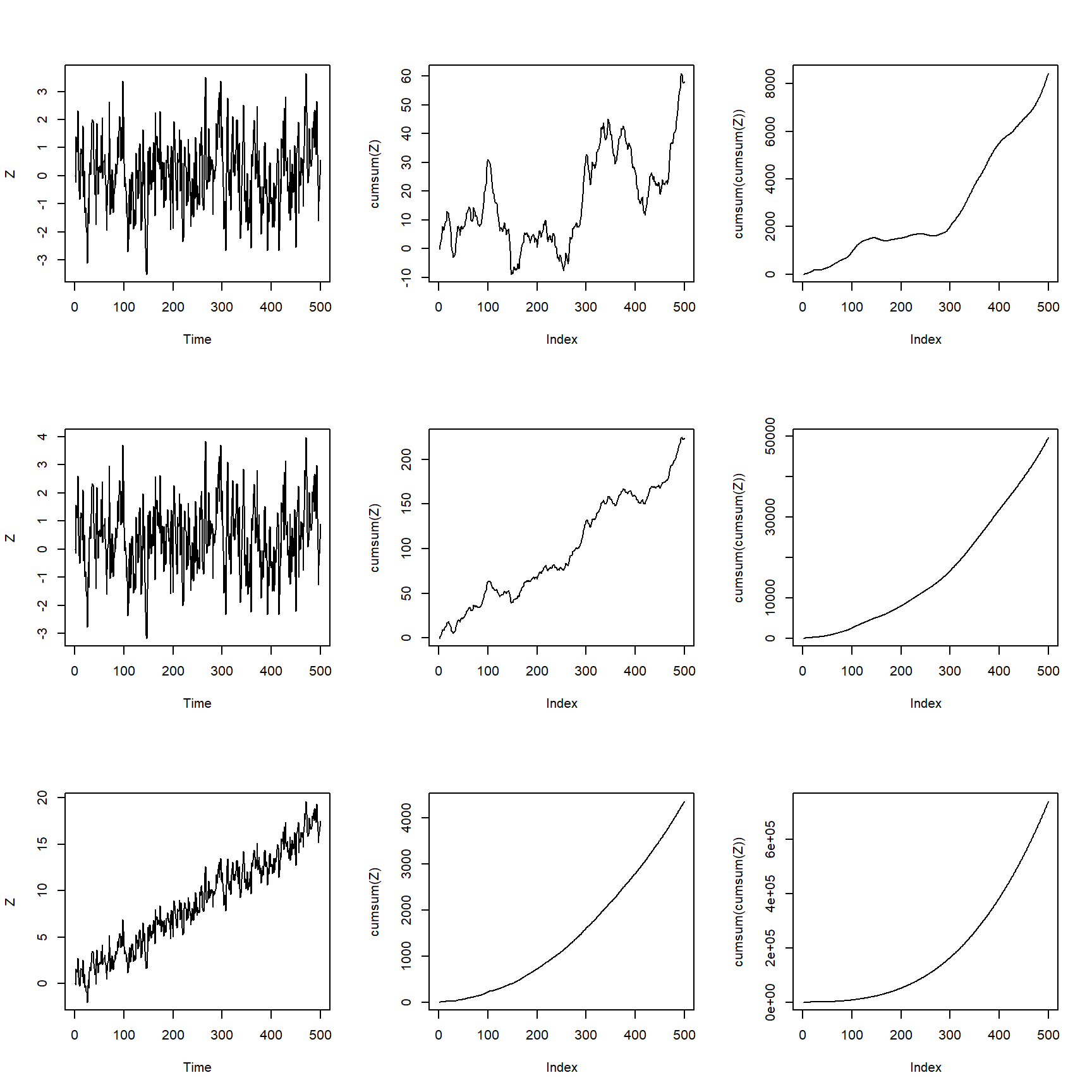# 5 Example of ARIMA Identification

Real Data Example: Here we analyze the seasonally adjusted electrical equipment orders data.

Here is the data

data("elecequip")
elecequip
##         Jan    Feb    Mar    Apr    May    Jun    Jul    Aug    Sep    Oct
## 1996  79.35  75.78  86.32  72.60  74.86  83.81  79.80  62.41  85.41  83.11
## 1997  78.64  77.42  89.86  81.27  78.68  89.51  83.67  69.80  91.09  89.43
## 1998  81.87  85.36  92.98  81.09  85.64  91.14  83.46  66.37  93.34  85.93
## 1999  81.59  81.77  91.24  79.45  86.99  96.60  97.99  79.13 103.56 100.89
## 2000  95.30  97.77 116.23 100.98 104.07 114.64 107.62  96.12 123.50 116.12
## 2001 100.56 103.05 119.06  92.46  98.75 111.14  96.13  79.72 102.07  96.18
## 2002  89.52  89.27 104.35  87.05  89.33 102.20  88.13  75.68  99.48  96.40
## 2003  89.34  86.91  98.90  85.54  85.25 101.14  91.80  76.98 104.33  99.72
## 2004  89.88  92.27 105.11  91.50  92.56 104.35  96.21  79.58 105.43  99.18
## 2005  91.65  90.56 105.52  92.18  91.22 109.04  99.26  83.36 110.80 104.95
## 2006  99.16  99.86 116.14 103.48 103.07 119.32 107.94  90.59 121.80 117.11
## 2007 103.93 104.10 125.72 104.70 108.45 123.11 108.89  94.07 121.88 116.81
## 2008 109.45 105.23 121.32 108.78 103.20 117.93 103.76  89.27 109.50 104.02
## 2009  77.38  75.19  86.40  74.13  74.10  85.61  79.90  65.36  88.09  84.60
## 2010  79.28  78.74  94.62  84.66  85.20 103.94  89.87  78.14  96.50  94.68
## 2011  92.57  89.16 104.48  89.45  93.40 102.90  93.77  77.58  95.04  91.77
## 2012  86.44  85.04  97.80
##         Nov    Dec
## 1996  84.21  89.70
## 1997  91.04  92.87
## 1998  86.81  93.30
## 1999  99.40 111.80
## 2000 116.86 128.61
## 2001 101.26 109.85
## 2002  96.16 101.00
## 2003 101.06 109.00
## 2004  99.77 113.55
## 2005 107.07 114.40
## 2006 113.71 120.37
## 2007 115.87 127.14
## 2008 100.12 101.18
## 2009  88.09 102.52
## 2010 101.77 103.48
## 2011  93.37  98.34
## 2012

We first remove the seasonality and visualize the data.

elecequip %>% stl(s.window='periodic') %>% seasadj() -> eeadj
autoplot(eeadj)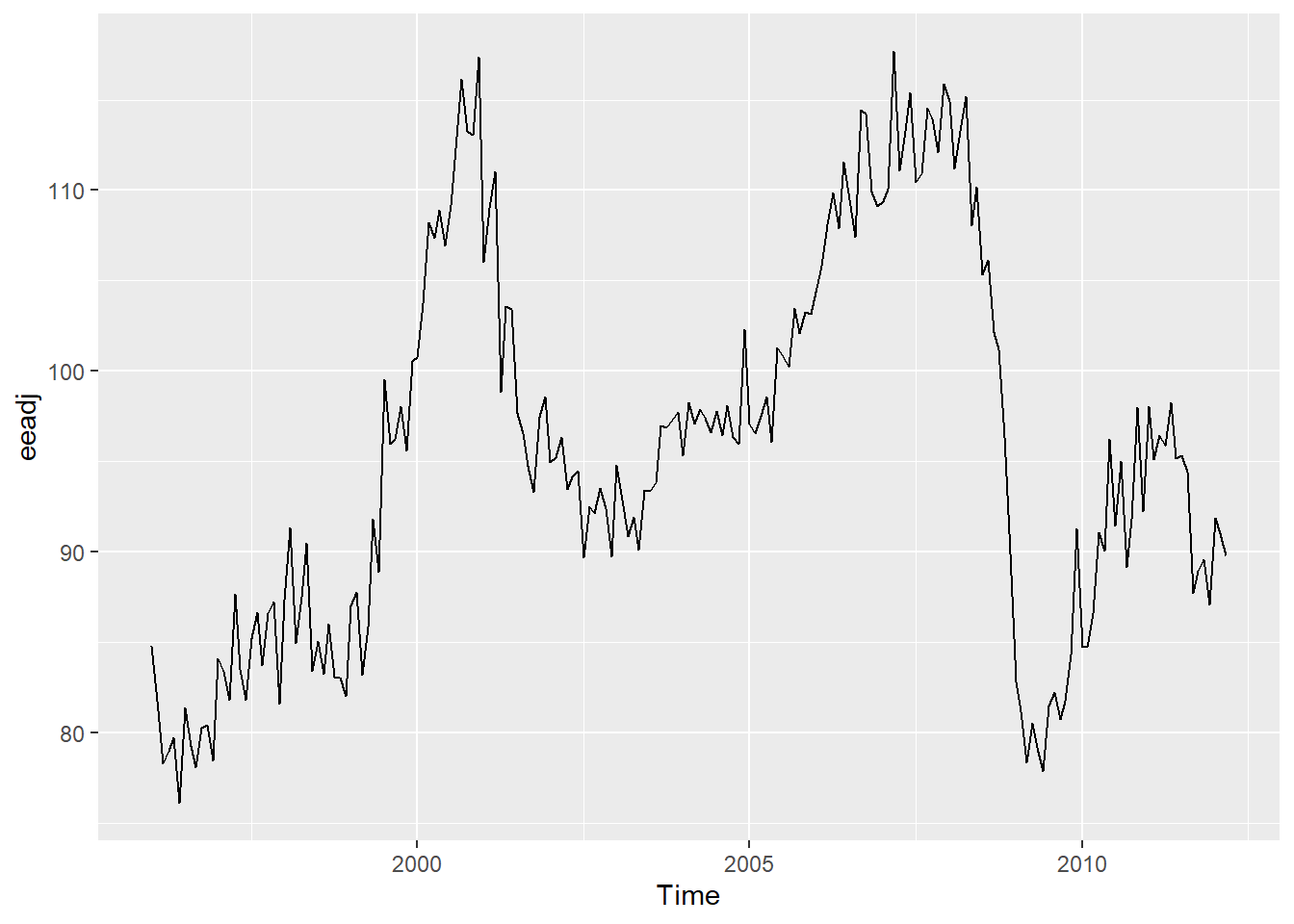We further perform ADF test to determine the stationarity. However, it is easy to see that it is not stationary.

adf.test(eeadj)
##
##  Augmented Dickey-Fuller Test
##
## Dickey-Fuller = -2.4033, Lag order = 5, p-value = 0.4073
## alternative hypothesis: stationary
test=ur.df(eeadj,type = "trend",lags = 5)
summary(test)
##
## ###############################################
## # Augmented Dickey-Fuller Test Unit Root Test #
## ###############################################
##
## Test regression trend
##
##
## Call:
## lm(formula = z.diff ~ z.lag.1 + 1 + tt + z.diff.lag)
##
## Residuals:
##     Min      1Q  Median      3Q     Max
## -9.0441 -1.8462 -0.1288  1.8738  9.4675
##
## Coefficients:
##              Estimate Std. Error t value Pr(>|t|)
## (Intercept)  5.619299   2.182805   2.574   0.0108 *
## z.lag.1     -0.056470   0.023496  -2.403   0.0173 *
## tt          -0.001179   0.004362  -0.270   0.7872
## z.diff.lag1 -0.357279   0.073859  -4.837 2.80e-06 ***
## z.diff.lag2 -0.029454   0.078309  -0.376   0.7073
## z.diff.lag3  0.369653   0.075122   4.921 1.93e-06 ***
## z.diff.lag4  0.124263   0.079551   1.562   0.1200
## z.diff.lag5  0.025847   0.073969   0.349   0.7272
## ---
## Signif. codes:  0 '***' 0.001 '**' 0.01 '*' 0.05 '.' 0.1 ' ' 1
##
## Residual standard error: 3.087 on 181 degrees of freedom
## Multiple R-squared:  0.2655, Adjusted R-squared:  0.2371
## F-statistic: 9.348 on 7 and 181 DF,  p-value: 7.152e-10
##
##
## Value of test-statistic is: -2.4033 2.3024 3.3939
##
## Critical values for test statistics:
##       1pct  5pct 10pct
## tau3 -3.99 -3.43 -3.13
## phi2  6.22  4.75  4.07
## phi3  8.43  6.49  5.47
test=ur.df(eeadj,type = "drift",lags = 5)
summary(test)
##
## ###############################################
## # Augmented Dickey-Fuller Test Unit Root Test #
## ###############################################
##
## Test regression drift
##
##
## Call:
## lm(formula = z.diff ~ z.lag.1 + 1 + z.diff.lag)
##
## Residuals:
##     Min      1Q  Median      3Q     Max
## -9.0821 -1.8463 -0.1465  1.8981  9.4675
##
## Coefficients:
##             Estimate Std. Error t value Pr(>|t|)
## (Intercept)  5.67679    2.16688   2.620  0.00954 **
## z.lag.1     -0.05830    0.02244  -2.598  0.01015 *
## z.diff.lag1 -0.35521    0.07327  -4.848 2.67e-06 ***
## z.diff.lag2 -0.02698    0.07757  -0.348  0.72839
## z.diff.lag3  0.37307    0.07386   5.051 1.06e-06 ***
## z.diff.lag4  0.12724    0.07858   1.619  0.10713
## z.diff.lag5  0.02785    0.07341   0.379  0.70490
## ---
## Signif. codes:  0 '***' 0.001 '**' 0.01 '*' 0.05 '.' 0.1 ' ' 1
##
## Residual standard error: 3.08 on 182 degrees of freedom
## Multiple R-squared:  0.2652, Adjusted R-squared:  0.241
## F-statistic: 10.95 on 6 and 182 DF,  p-value: 2.108e-10
##
##
## Value of test-statistic is: -2.5979 3.4345
##
## Critical values for test statistics:
##       1pct  5pct 10pct
## tau2 -3.46 -2.88 -2.57
## phi1  6.52  4.63  3.81
test=ur.df(eeadj,type = "none",lags = 5)
summary(test)
##
## ###############################################
## # Augmented Dickey-Fuller Test Unit Root Test #
## ###############################################
##
## Test regression none
##
##
## Call:
## lm(formula = z.diff ~ z.lag.1 - 1 + z.diff.lag)
##
## Residuals:
##     Min      1Q  Median      3Q     Max
## -9.0209 -1.7146 -0.1254  1.9183  9.2691
##
## Coefficients:
##               Estimate Std. Error t value Pr(>|t|)
## z.lag.1      0.0001757  0.0023590   0.074    0.941
## z.diff.lag1 -0.3781195  0.0739069  -5.116 7.83e-07 ***
## z.diff.lag2 -0.0385448  0.0786792  -0.490    0.625
## z.diff.lag3  0.3511470  0.0745555   4.710 4.89e-06 ***
## z.diff.lag4  0.0917809  0.0786379   1.167    0.245
## z.diff.lag5 -0.0021925  0.0736622  -0.030    0.976
## ---
## Signif. codes:  0 '***' 0.001 '**' 0.01 '*' 0.05 '.' 0.1 ' ' 1
##
## Residual standard error: 3.129 on 183 degrees of freedom
## Multiple R-squared:  0.2379, Adjusted R-squared:  0.2129
## F-statistic: 9.519 on 6 and 183 DF,  p-value: 4.218e-09
##
##
## Value of test-statistic is: 0.0745
##
## Critical values for test statistics:
##       1pct  5pct 10pct
## tau1 -2.58 -1.95 -1.62
test=ur.kpss(eeadj)
summary(test)
##
## #######################
## # KPSS Unit Root Test #
## #######################
##
## Test is of type: mu with 4 lags.
##
## Value of test-statistic is: 0.7017
##
## Critical value for a significance level of:
##                 10pct  5pct 2.5pct  1pct
## critical values 0.347 0.463  0.574 0.739

The results are consistent with our judgement. Therefore, we take the 1st order difference and check again

eeadj %>% diff() %>% adf.test()
## Warning in adf.test(.): p-value smaller than printed p-value
##
##  Augmented Dickey-Fuller Test
##
## data:  .
## Dickey-Fuller = -4.2461, Lag order = 5, p-value = 0.01
## alternative hypothesis: stationary

The differenced series is stationary. We plot its ACF and PACF.

eeadj %>% diff() %>% ggtsdisplay(main="")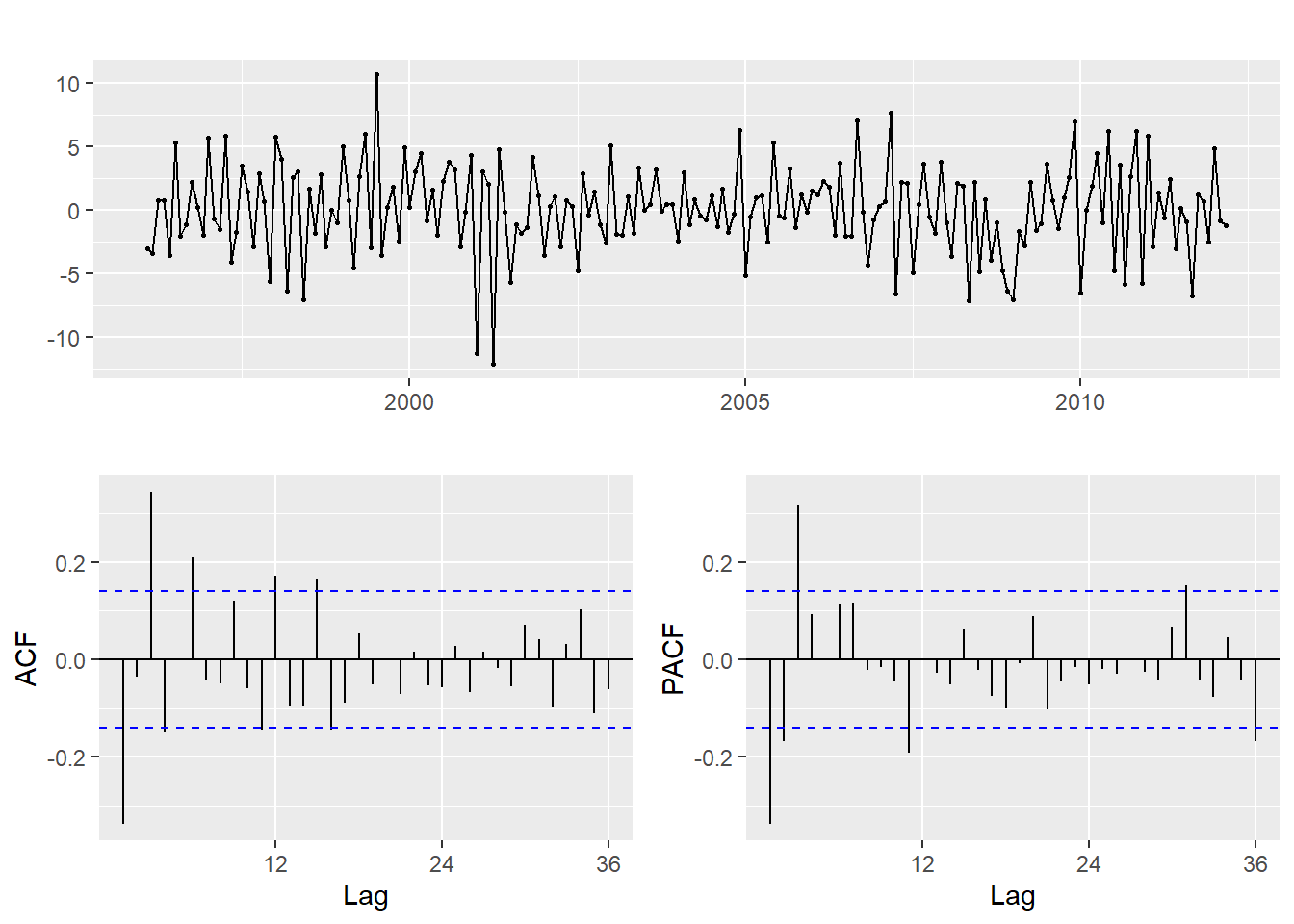The PACF is suggestive of an AR(3) model. So an initial candidate model is an ARIMA(3,1,0). There are no other obvious candidate models.

We fit an ARIMA(3,1,0) model along with variations including ARIMA(4,1,0), ARIMA(2,1,0), ARIMA(3,1,1), etc.

(fit <- Arima(eeadj, order=c(3,1,0)))
## Series: eeadj
## ARIMA(3,1,0)
##
## Coefficients:
##           ar1      ar2     ar3
##       -0.3418  -0.0426  0.3185
## s.e.   0.0681   0.0725  0.0682
##
## sigma^2 estimated as 9.639:  log likelihood=-493.8
## AIC=995.6   AICc=995.81   BIC=1008.67
checkresiduals(fit)